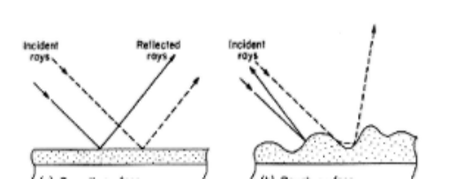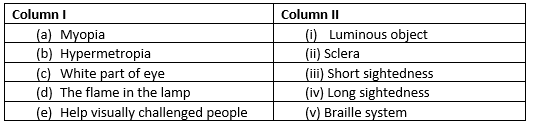# Light Extra Questions for Class 8

In this page we have Extra questions on light class 8 . Hope you like them and do not forget to like , social shar and comment at the end of the page.

Question 1
(i)What is the difference between luminous and non-luminous?
(ii)Explain the given term “Angle of Incidence”.
(iii)What is the regular reflection of light?
(iv)Name the colours present in the white light?
(v)Define Tactual aids.

Question 2
Multiple Choice Questions (a) Which type of reflection shown by given figure:(i)Angle of incidence
(ii)Regular and irregular
(ii)Law of reflection
(iv)Angle of reflection

(b)What is the value of $\theta$,if n=5?
(i)80
(ii)72
(iii)89
(iv)70

(c)Which device is used to see things above the surface of the water?
(i)Kaleidoscope
(ii)Periscope
(iii)spectrum
(iv)mirror

(d)The light sensitive layers of nerve tissue at the back of the eye that receive images and sends them as electric signals through the optic nerve to the brain.
(i)Iris
(ii)Cornea
(iii)Pupil
(iv)Retina

(e)What is the minimum distance which our normal eye can see distinctly?
(i)20 cm
(ii)28 cm
(iii)25 cm
(iv)All of the above

Question 3
Match the column:Question 4
What is the law of reflection?

Question 5
Explain the types of reflection?

Question 6
Briefly describe kaleidoscope.

Question 7
Why Ambulance is written opposite on the Ambulance?

Question 8
What is periscope? Write its uses.

Question 9
Write the definition of power of accommodation.

Question 10
What happens when we see a faraway object?

Question 11
What is cataract and how it affects the eye?

Question 12
Describe multiple reflection with the help of a suitable diagram.

Question 13
Write a short note on Braille system.

• Notes
• Assignments
• NCERT Solution

Latest Articles
Synthetic Fibres and Plastics Class 8 Practice questions

Class 8 science chapter 5 extra questions and Answers

Mass Calculator

3 Fraction calculator

Garbage in Garbage out Extra Questions7